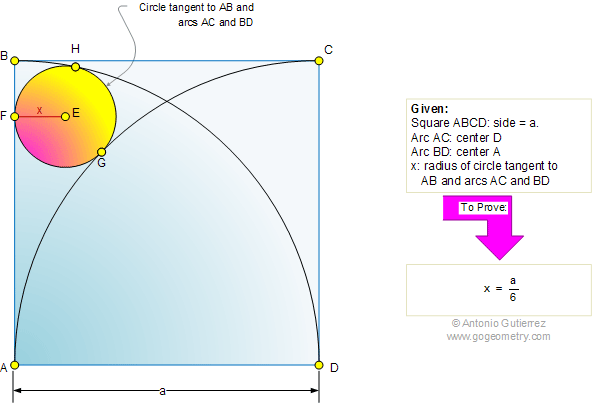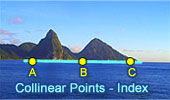# Geometry Problem 462: Square, Arcs, 90 Degrees, Circle, Tangent, Radius, Measurement

 The figure shows a square ABCD of side a. It contains arcs BD and AC drawn with centers at A and D, respectively. If x is the radius of circle tangent to AB and arcs AC and BD, prove that x = a/6.More Problems: Proposed Problem 463. Three Concentric Circles, Secant, Tangent, Triangle, Area. Proposed Problem 461. Three Circles, Tangent, Right Angle, Center, Distance, Measurement. Proposed Problem 460. Circle, Tangent, Perpendicular, Radius, Distance, Measurement. Proposed Problem 459. Right triangle, Squares, Distance, Measurement.Home | Search | Geometry | Problems | All Problems | Visual Index | 461-470 | Circle Inscribed in a Square | Email | Solution / comment | by Antonio Gutierrez